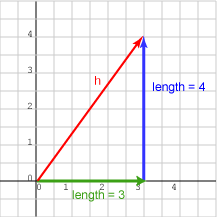### Answer:

a = (0,4)T is aligned with the Y axis. Its length, 4 units, can be read off the diagram.

Of course, vectors have no fixed location, so vector a could have been drawn anywhere and would have the same length.

# Pythagorean FormulaThe diagram shows the vectors of length 3 and 4, and with a new vector, h.

The length of vector h is harder to figure out. But not much harder, especially if you know about "3, 4, 5 right triangles." The three vectors can be arranged into a right triangle with h as the hypotenuse. The other sides are 3 and 4, so the length of h is 5.

You probably know the Pythagorean Formula:

 ```(length of hypotenuse)2   =   (length of first side)2   +   (length of second side)2 ```

Using this with a right triangle with sides of 3 and 4:

 ```(length of hypotenuse)2   =   32 + 42 (length of hypotenuse)2   =   9 + 16 = 25 (length of hypotenuse)   =   5 ```

### QUESTION 3:

What is the length of the hypotenuse of a right triangle whose two sides are 6 and 8?